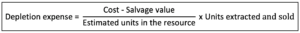# Calculate Depletion Expense

## Overview

In accounting, the company usually uses the units-of-activity method to calculate the depletion expense. This is due to the actual extracted resources usually fairly represents the exhaustion in each period of a natural resource that the company has invested in.

The units-of-activity method is the measurement that uses the units of activity during the year to apply the cost per unit in order to determine the annual expense. This is why it is suitable to use in the calculation of depletion expense as the available natural resource will be reduced by the number of extracted units in each period.

## Depletion expense formula

The company can calculate the depletion expense with the formula of using the total cost to deduct the salvage value and dividing the result with the total estimated units in the resource to get the cost per unit. Then, it can multiply the cost per unit with the units extracted and sold to get the depletion expense in the period.This depletion expense formula is the units-of-activity method where the depletion represents the exhaustion of a natural resource. Likewise, this formula functions by using the actual units extracted to reflect the depletion or the exhaustion of a natural resource in each period.

## Example of depletion expense calculation

For example, on January 1, 2020, the company ABC has invested \$2,000,000 in a coal mine that is estimated to contain 1,000,000 tons of coal. The mine is expected to be able to sell for \$100,000 at the end of its useful life and the company ABC has extracted and sold 120,000 tons of coal in 2020.

Calculate the depletion expense in 2020.

Solution:

With the information in the example above, we can calculate the depletion expense as below:

Depletion expense = (2,000,000 – 100,000) / 1,000,000 x 120,000 = \$228,000

In this case, with the result of the calculation, the depletion expense in 2020 is \$228,000. Likewise, the company ABC can report the \$228,000 in the income statement of 2020 as the depletion expense.

It is important to note that the depletion expense should be recorded in the period that the extracted natural resource (e.g. coal) is sold. The unsold part of the extracted natural resource should be recorded as inventory.